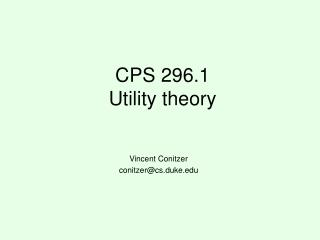Download PresentationCPS 296.1 Utility theory

# CPS 296.1 Utility theory - PowerPoint PPT PresentationDownload Presentation## CPS 296.1 Utility theory

- - - - - - - - - - - - - - - - - - - - - - - - - - - E N D - - - - - - - - - - - - - - - - - - - - - - - - - - -
##### Presentation Transcript

1. CPS 296.1Utility theory Vincent Conitzer conitzer@cs.duke.edu

2. Risk attitudes • Which would you prefer? • A lottery ticket that pays out \$10 with probability .5 and \$0 otherwise, or • A lottery ticket that pays out \$3 with probability 1 • How about: • A lottery ticket that pays out \$100,000,000 with probability .5 and \$0 otherwise, or • A lottery ticket that pays out \$30,000,000 with probability 1 • Usually, people do not simply go by expected value • An agent is risk-neutral if she only cares about the expected value of the lottery ticket • An agent is risk-averse if she always prefers the expected value of the lottery ticket to the lottery ticket • Most people are like this • An agent is risk-seeking if she always prefers the lottery ticket to the expected value of the lottery ticket

3. Decreasing marginal utility • Typically, at some point, having an extra dollar does not make people much happier (decreasing marginal utility) utility buy a nicer car (utility = 3) buy a car (utility = 2) buy a bike (utility = 1) money \$200 \$1500 \$5000

4. Maximizing expected utility utility buy a nicer car (utility = 3) • Lottery 1: get \$1500 with probability 1 • gives expected utility 2 • Lottery 2: get \$5000 with probability .4, \$200 otherwise • gives expected utility .4*3 + .6*1 = 1.8 • (expected amount of money = .4*\$5000 + .6*\$200 = \$2120 > \$1500) • So: maximizing expected utility is consistent with risk aversion buy a car (utility = 2) buy a bike (utility = 1) money \$200 \$1500 \$5000

5. Different possible risk attitudes under expected utility maximization utility • Green has decreasing marginal utility → risk-averse • Blue has constant marginal utility → risk-neutral • Red has increasing marginal utility → risk-seeking • Grey’s marginal utility is sometimes increasing, sometimes decreasing → neither risk-averse (everywhere) nor risk-seeking (everywhere) money

6. What is utility, anyway? • Function u: O →  (O is the set of “outcomes” that lotteries randomize over) • What are its units? • It doesn’t really matter • If you replace your utility function by u’(o) = a + bu(o), your behavior will be unchanged • Why would you want to maximize expected utility? • This is a question about preferences over lotteries

7. Compound lotteries • For two lottery tickets L and L’, let pL + (1-p)L’ be the “compound” lottery ticket where you get lottery ticket L with probability p, and L’ with probability 1-p pL+(1-p)L’ pL+(1-p)L’ p=50% 1-p=50% = L L’ 25% 12.5% 50% 12.5% 75% 25% o1 o2 o3 o4 50% 25% 25% o1 o2 o3 o2 o4

8. Sufficient conditions for expected utility • L ≥ L’ means that L is (weakly) preferred to L’ • (≥ should be complete, transitive) • Expected utility theorem. Suppose • (continuity axiom) for all L, L’, L’’, {p: pL + (1-p)L’ ≥ L’’} and {p: pL + (1-p)L’ ≤ L’’} are closed sets, • (independence axiom – more controversial) for all L, L’, L’’, p, we have L ≥ L’ if and only if pL + (1-p)L’’ ≥ pL’ + (1-p)L’’ then there exists a function u: O →  so that L ≥ L’ if and only if L gives a higher expected value of u than L’